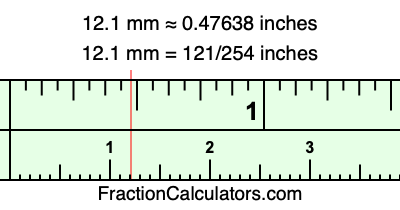12.1 mm in inchesHere is how to convert 12.1 mm to inches. We will give you the fractional answer, the decimal answer, and illustrate the answer on our tape measure.

One millimeter is equal to 5/127 inches, and 12.1 as a fraction is 121/10. Therefore, to get the fractional answer to 12.1 mm in inches, we multiply 121/10 by 5/127 and simplify if necessary.

cm × 5/127 = inches
121/10 × 5/127 = inches
121/10 × 5/127 = 121/254
121/10 mm = 121/254 inches
12.1 mm = 121/254 inches

As promised above, we also have the answer to 12.1 mm in inches in decimal form. Although the fractional answer above is exact, the decimal answer may be rounded if necessary:

121/254 ≈ 0.476377952755906
121/254 ≈ 0.47638
12.1 mm ≈ 0.47638 inches

Our image below shows 12.1 mm on a tape measure. The top row of the tape measure is inches, and each mark on the bottom row is 1 millimeter (10 millimeter marks equal 1 centimeter).As you can see, we drew a red line where 12.1 mm and 0.47638 inches meet on the measuring tape.

Millimeters to Inches Calculator
Here you can convert another length in millimeters to inches.

Convert  mm to inches.

What is 12.2 mm in inches?
Here is another inches to millimeters calculation that we have done for you.